Graphing a Cube Root Function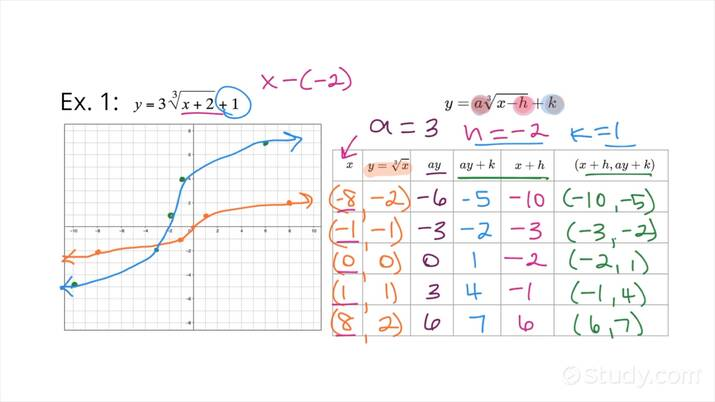An error occurred trying to load this video.

Try refreshing the page, or contact customer support.

• 00:04 Graphing a cube root…
• 03:54 Graphing a cube root…
Marisa Lettini, Lynn Ellis
• Instructors
Marisa Lettini

Marisa Lettini has taught various courses in mathematics ranging from Algebra 1 to Precalculus for over 11 years. She has earned a BS and MS in Industrial Engineering from NJIT. Marisa currently has her teaching certificate in the State of New Jersey.

•
Lynn Ellis

Lynn Ellis has taught mathematics to high school and community college students for over 13 years. She has a Bachelor's degree in Mathematics from Middlebury College and a Master's Degree in Education from the University of Phoenix.

Graphing a Cube Root Function

Step 1: Graph the parent function by creating a table.

Step 2: Multiply the y-values by a.

Step 3. Add/subtract the x-values by h and add/subtract the new y-values by k.

Step 4: Plot the new coordinates and graph the translated/transformed function.

Graphing a Cube Root Function Vocabulary

Parent function: The simplest function of a family of functions, given in the form {eq}f(x)=\sqrt{x} {/eq}

The graph and table below represent the parent function.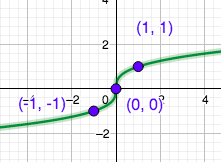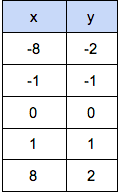The function translation/transformation rules:

The Translated/Transformed Function is in the form {eq}g(x) =a\sqrt{x-h} +k {/eq}

a = Vertical Stretch or Compression.

If a > 1, then it is a Vertical Stretch.

For example, {eq}g(x)=2\sqrt{x} {/eq} is a Vertical Stretch by 2.

If 0 < a < 1, then it is a Vertical Compression.

For example, {eq}g(x)=\frac{1}{2}\sqrt{x} {/eq} is a Vertical Compression by {eq}\frac{1}{2} {/eq}

The following graph compares the Parent Function with a Vertical Stretch by 2 and a Vertical Compression by

{eq}\frac{1}{2} {/eq}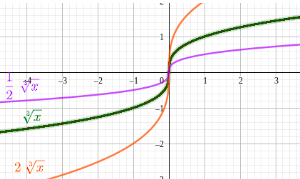h = horizontal translation

If h > 0 ,then it is a slide to the right

For example, {eq}g(x)=\sqrt{x-3} {/eq} is a slide to the right by 3 units.

If h < 0, then it is a slide to the left.

For example, {eq}g(x)=\sqrt{x+3} {/eq} is a slide to left by 3 units

The following graph compares the Parent Function with a Horizontal Slide to right 3 units and a Horizontal Slide to the left 3 units.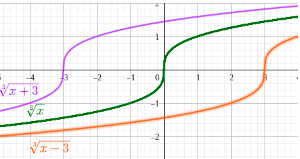k = vertical translation

If k > 0, then it is a slide up.

For example, {eq}g(x)=\sqrt{x}+2 {/eq} is a slide up 2 units

If k < 0, then it is a slide down.

For example, {eq}g(x)=\sqrt{x}-2 {/eq} is a slide down 2 units

The following graph compares the Parent Function with a Vertical Slide up 2 units and a Vertical Slide down 2 units.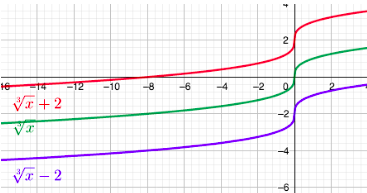Now let's look at two examples of how to graph a Cube Root Function:

How to Graph a Cubic Root Function Example 1

Graph {eq}g(x)=3\sqrt{x+4}+2 {/eq}

Step 1: Graph the parent function by creating a table.Step 2: Multiply the y-values by the value of a, which is 3.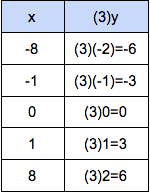Step 3: Subtract the x-values by h, which is 4, and add the new y-values by k, which is 2.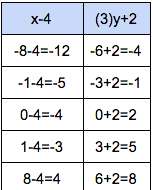Step 4: Plot the new coordinates and graph the translated/transformed function.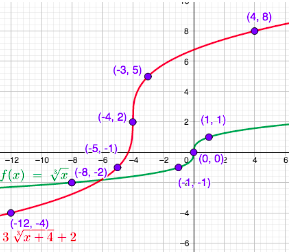How to Graph a Cubic Root Function Example 2

Choose the graph of the function{eq}g(x)=4\sqrt{x-3}-2 {/eq}

Step 1: Graph the Parent Function by creating a table.

Step 2: Multiply the y-values by a, which is 4.

Step 3: Add the x-values by h, which is 3, and subtract the new y-values by k, which is 2.

Step 4: Plot the new coordinates and graph the translated/transformed function.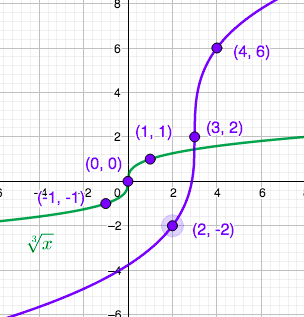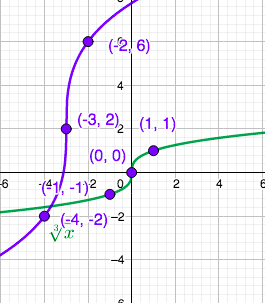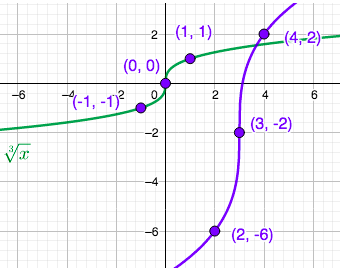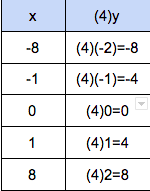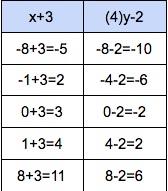The correct answer is Graph 3.